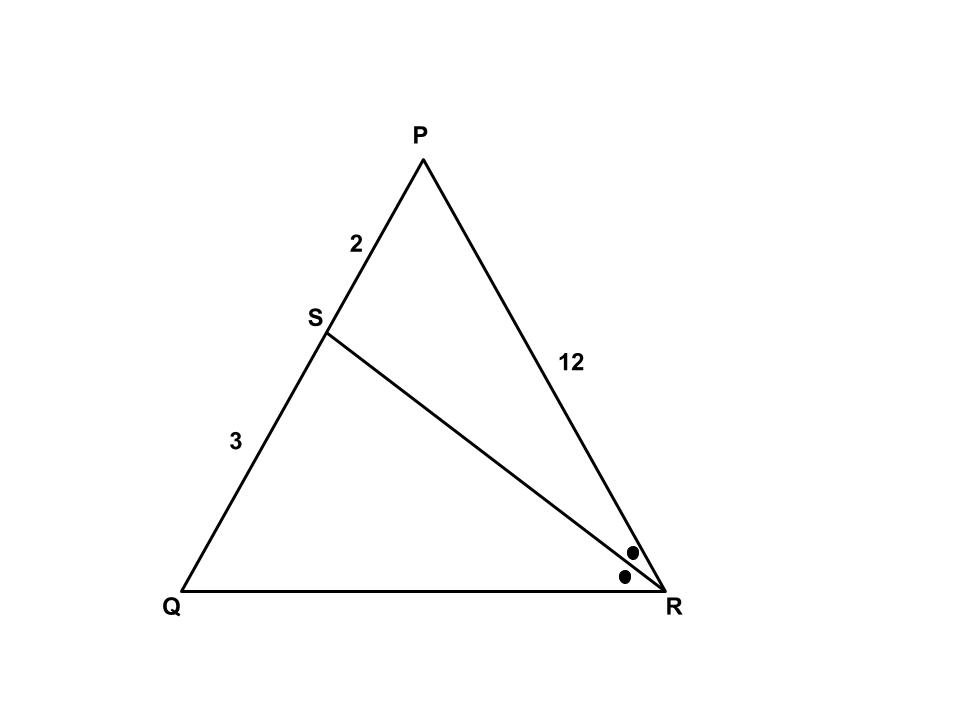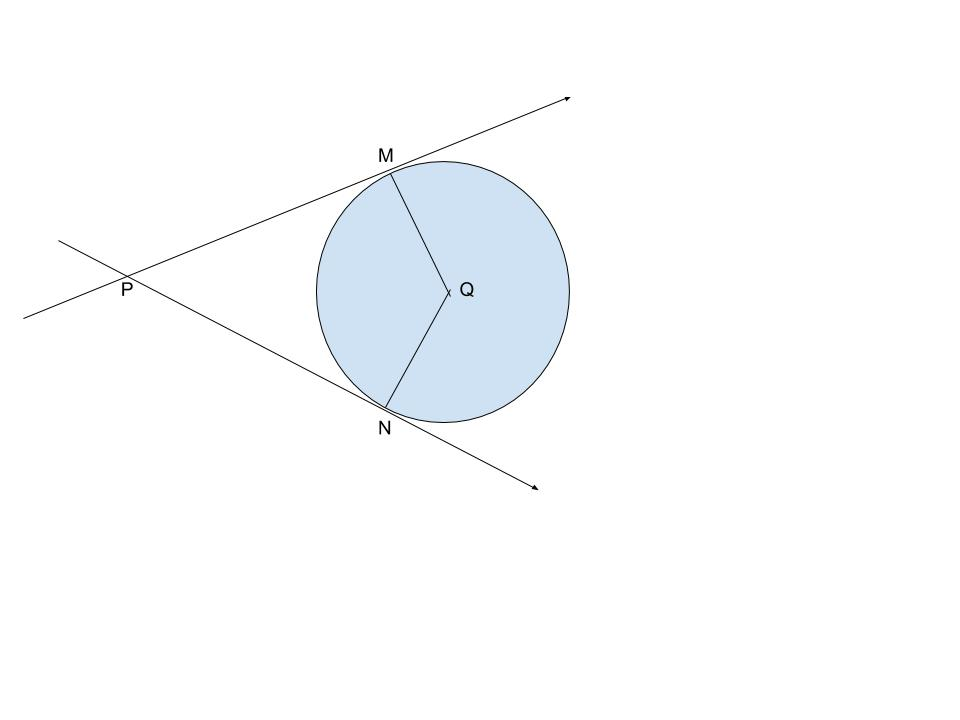SSC BOARD PAPERS IMPORTANT TOPICS COVERED FOR BOARD EXAM 2024

### GEOMETRY PRELIMINARY PAPER 2016

OMTEX CLASSES THE HOME OF SUCCESS.
GEOMETRY PRELIMINARY PAPER 2016

1. Solve any five of the sub – questions: 

(i) The terminal arm is in quadrant II, what are the possible angles?
(ii) Find the slope and y - intercept of the line y = - 2x + 3.
(iii) Find the slope of the line whose inclination is θ = 300.
(iv) Find the diagonal of a square whose side is 10 cm.
(v) If the angle θ = - 600, find the value of sin θ .
(vi) If two circles with radii 5 cm and 3 cm respectively touch internally, find the distance between their centres.

2. Solve any four sub - questions: 

(i) If sin θ = 5/13, where θ is an acute angle, find the value of cos θ.
(ii) Draw ∠ABC of Measure 1150 and bisect it.
(iii) Find the slope of the line passing through the points C (3 , 5) and D ( - 2, - 3 )
(iv) Find the area of the sector whose arc length and radius are 10 cm and 5 cm respectively.
(v) Find the total surface area of a cube with side 1 metre.
(vi) In the following figure, In triangle PQR, seg RS is the bisector of Angle PRQ. If PS = 2, SQ = 3, PR = 12, find QR.3. Solve any three sub - questions: 
(i) In the following figure, Q is the centre of a circle and PM, PN are tangent segments to the circle. If  ∠ MPN = 400, find ∠ MQN.(ii) Draw the tangents to the circle from the point L with radius 2.8 cm. Point ‘L’ is at a distance 7 cm from the centre ‘M’.
(iii) The ratio of the areas of two triangles with the common base is 6:5. Height of the larger triangle is 9 cm, then find the corresponding height of the smaller triangle.
(iv) Two buildings are in front of each other on either side of a road of width 10 metres. From the top of the first building which is 30 metres high, the angle of elevation to the top of the second is 450. What is the height of the second building?
(v) Find the length of the arc of a circle with radius 0.7m and area of the sector is 0.49 sq. m.
(vi) Prove that ‘the opposite angles of a cyclic quadrilateral are supplementary”.

4. Solve any two sub – questions: 
(i) If a line parallel to one side of a triangle intersects the other two sides in two distinct points, then the other two sides are divided in the same ratio by it.
(ii) Draw the circumcircle of Triangle PMT in which PM = 5.4 cm, Angle P = 600, Angle M = 700.
(iii) Using the slope concept, check whether the following points are collinear or not. A(7 , 8) , B(-5 , 2) and C (3 , 6)

5. Solve any two sub – questions: 
(i) Prove that: In a right-angled triangle, the square of the hypotenuse is equal to the sum of the squares of the other two sides.
(ii) If cos θ = 7/25 and θ is in fourth quadrant, find the other five trigonometric ratios.
(iii) ∆ RST ∼ ∆ UAY. In ∆ RST, RS = 6 cm, ∠ S = 500, ST = 7.5 cm. The corresponding sides of ∆ RST and ∆ UAY are in the ratio 5 : 4. Construct ∆ UAY.